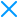# Variable Grouping

Potency Low
Resilience High
Cost Low
Tags: variables, grouping, hoisting, obfuscation

## Description

Variable Grouping groups your variable declarations into a single variable statement list. Additionally, variable hoisting is performed in optimization-time. Hoisting is JavaScript default behavior of moving declarations to the top of the scope. This behavior gives you the ability to declare a function or a variable after it is used.

### Code Annotation Example

``// @jscrambler enable variableGrouping``

## Examples

The following contrived example does nothing besides declaring a bunch of variables in different scopes:

```var a = 1;
var b = 2;
(function () {
var a = 1;
var b = 2;
var c = function () {
var a = 1;
var b = 2;
var c = 3;
}();
var d = 2;
function e() {}
var f = 1;
var g = 2;
}());
var f = 1;
var g = 2;```

The resulting code looks like this:

```var a, b, f, g;
a = 1;
b = 2;
(function () {
var a, b, c, d, f, g;
a = 1;
b = 2;
c = function () {
var a, b, c;
a = 1;
b = 2;
c = 3;
}();
d = 2;
function e() {}
f = 1;
g = 2;
}());
f = 1;
g = 2;```

## Browser Compatibility

Browser Compatible Versions Tested Versions Notes
Chrome 50+ 50+
Firefox 40+ 40+
Internet Explorer 8+ 8+
Microsoft Edge 18+ 18+
Safari 9.1+ 9.1+

## API Parameters

Example:

```{
"keys": {
"accessKey": "XXXXXX",
"secretKey": "YYYYYY"
},
"applicationId": "ZZZZZZ",
"params": [
{
"name": "variableGrouping"
}
]
}```